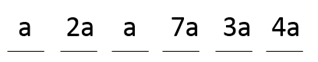# CAT 2019 Question Paper | Quants Slot 2

###### CAT Previous Year Paper | CAT Quants Questions | Question 26

It is very common for CAT Aspirants to get stuck in a question that they thought they could easily steer through one after the other. To break this cycle, it is important to practice a lot of questions from various topics. Practicing CAT previous year paper helps as it helps you break the loop of getting stuck with questions by making you go through the actual CAT questions. So do not wait, dive right into solving these questions.
If you are looking for more questions, visit 2IIM's CAT Question Bank.

Question 26 : In a six-digit number, the sixth, that is, the rightmost, digit is the sum of the first three digits, the fifth digit is the sum of first two digits, the third digit is equal to the first digit, the second digit is twice the first digit and the fourth digit is the sum of fifth and sixth digits. Then, the largest possible value of the fourth digit is [TITA]

## Best CAT Coaching in Chennai

#### CAT Coaching in Chennai - CAT 2022Limited Seats Available - Register Now!Let the first digit be = a
Second digit = Twice of first digit = 2a
Third digit = First digit = a
Fifth digit = Sum of first two digits = a + 2a = 3a
Sixth digit = Sum of first three digits = a + 2a + a = 4a
Fourth digit = Sum of fifth and sixth digit = 3a + 4a = 7a

Largest possible value of fourth digit = 7 (any more than that would be > 10 and not satisfy the condition)

The question is "In a six-digit number, the sixth, that is, the rightmost, digit is the sum of the first three digits, the fifth digit is the sum of first two digits, the third digit is equal to the first digit, the second digit is twice the first digit and the fourth digit is the sum of fifth and sixth digits. Then, the largest possible value of the fourth digit is [TITA]"

##### Hence, the answer is 7

###### CAT Coaching in ChennaiCAT 2023

Classroom Batches Starting Now! @Gopalapuram

###### Best CAT Coaching in Chennai Introductory offer of 5000/-

Attend a Demo Class

##### Where is 2IIM located?

2IIM Online CAT Coaching
A Fermat Education Initiative,
58/16, Indira Gandhi Street,
Kaveri Rangan Nagar, Saligramam, Chennai 600 093

##### How to reach 2IIM?

Mobile: (91) 99626 48484 / 94459 38484
WhatsApp: WhatsApp Now
Email: info@2iim.com